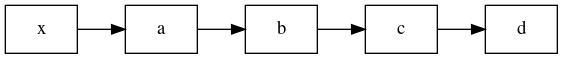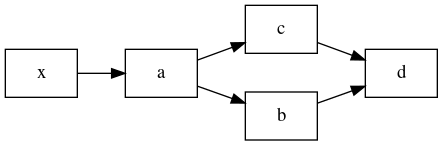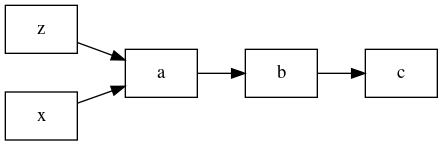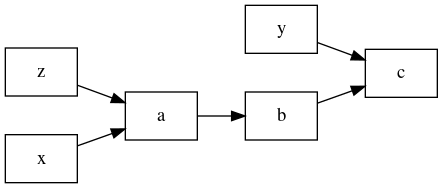A small toolkit for building dataflow-based framework.

# CRayGraph

A toolkit for defining dataflow-based frameworks. Intended for use in CRayNN (network definitions) and CRayFlow (dataflow definitions).

## Installation

### via PyPi

pip install craygraph


### via git

CRayGraph can be installed directly from gitlab.com:

pip install git+https://gitlab.com/craynn/craygraph.git


however, as repository updates quite often, it is recommend to clone the repository and install the package in development mode:

git clone git@gitlab.com:craynn/craygraph.git
cd craygraph/
pip install -e .


## Usage

This package provides three main functions:

• cragraph.graph : utilities for constructing easily readable definitions of directed acyclic graphs (DAGs);
• cragraph.meta : functions that help quickly adopt custom classes to work with cragraph.graph.

## DAG definition language

The main feature of craygraph is intuitive and readable DAG definition language.

### Quick introduction

Below, functions like a, b, c, d represent node (subgraph) constructors, i.e. (*Node) -> Node or (*Node) -> list[Nodes], x, y, z represent input nodes.

#### Tuple of functions = composition

achain(a, b, c, d)(x)


is equivalent to:

d(c(b(a(x))))


and produces:#### List of functions = independent application:

result = achain(a, [b, c], d)(x)


is equivalent to:

a_x = a(x)
result = d(b(a_x), c(a_x))


and results in the following graph:craygraph.graph contains some helper functions. select[items](body) selects inputs according to items and applies body

select[item](f)(*args) == f(args[item])


for example:

result = achain(
select[0, -1](a, b),
c
)(x, y, z)


is equivalent to:

result = c(b(a(x, z)))


which results in (node y is omitted since it is not used):with_inputs[items](body) is similar to select, but it takes inputs and replaces selected inputs with the result of body:

result = achain(
with_inputs[0, -1](a, b),
c
)(x, y, z)


is equivalent to:

result = c(b(a(x, z)))


which results in:## Project details

This version0.3.00.2.1

Uploaded source